• +91 9971497814
• info@interviewmaterial.com

# RD Chapter 3- Pair of Linear Equations in Two Variables Ex-3.11 Interview Questions Answers

### Related Subjects

Question 1 : If in a rectangle, the length is increased and breadth reduced each by 2 units, the area is reduced by 28 square units. If, however the length is reduced by 1 unit and the breadth increased by 2 units, the area increased by 33 square units. Find the area of the rectangle.

Let the length of rectangle = x units
Area = Length x breadth = x x y = xy sq. units
According to the condition given,
(x + 2) (y – 2) = xy – 28
=> xy – 2x + 2y – 4 = xy – 28
=> -2x + 2y = -28 + 4 = -24
=> x – y = 12 ….(i)
(Dividing by -2)
and (x – 1) (y + 2) = xy + 33
=> xy + 2x – y – 2 = xy + 33
=> 2x – y = 33 + 2
=> 2x – y = 35 ….(ii)
Subtracting (i), from (ii)
x = 23
Substituting the value of x in (i)
23 – y = 12
=> -y = 12 – 23 = -11
=> y = 11
Area of the rectangle = xy = 23 x 11 = 253 sq. units

Question 2 : The area of a rectangle remains the same if the length is increased by 7 metres and the breadth is decreased by 3 metres. The area remains unaffected if the length is decreased by 7 metres and breadth is increased by 5 metres. Find the dimensions of the rectangle.

Let the length of a rectangle = x m
Area = Length x breadth = xy sq. m
According to the condition given,
(x + 7)(y – 3) = xy
=> xy – 3x + 7y – 21 = xy
-3x + 7y = 21 ….(i)
and (x – 7) (y + 5) = xy
=>xy + 5x – 7y = xy
5x – 7y = 35 …(ii)
(i) from (ii)
2x = 56
=> x = 28
Substituting the value of x in (i)
-3 x 28 + 7y = 21
– 84 +7y = 21
=> 7y = 21 + 84 = 105
y = 15
Length of the rectangle = 28 m and breadth = 15 m

Question 3 : In a rectangle, if the length is increased by 3 metres and breadth is decreased by 4 metres, the area of the rectangle is reduced by 67 square metres. If length reduced by 1 metre and breath increased by 4 metres, the area is increased by is 89 sq. metres. Find the dimensions of the rectangle.

Let length of rectangle = x m
Area = Length x breadth = xy m2
According to the given condition,
(x + 3) (y – 4) = xy – 67
=> xy – 4x + 3y – 12 = xy – 67
=>-4x + 3y = -67+ 12 = -55
=> 4x – 3y = 55 ….(i)
and (x -1) (y + 4) = xy + 89
=> xy + 4x – y – 4 = xy + 89
=> 4x – y = 89 + 4 = 93 ….(ii)
Subtracting (i) from (ii)
2y = 38
=> y = 19
Substituting the value of y in (i)
4x – 3 x 19 = 55
=> 4x – 57 = 55
=> 4x = 55 + 57 = 112
=> x = 28
Length of the rectangle = 28 m and breadth = 19 m

Question 4 : The incomes of X and Y are in the ratio of 8 : 7 and their expenditures are in the ratio 19 : 16. If each saves ₹ 1250, find their incomes.

Incomes of X and Y are in the ratio 8 : 7
and their expenditures = 19 : 16
Let income of X = ₹ 8x
and income of Y = ₹ 7x
and let expenditures of X = 19y
and expenditure of Y = 16y
Saving in each case is save i.e. ₹ 1250
8x – 19y = 1250 ….(i)
and 7x – 16y = 1250 ….(ii)
Multiplying (i) by 7 and (ii) by 8, we get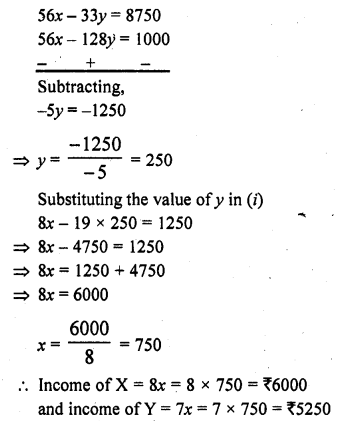Question 5 : A and B each has some money. If A gives ₹ 30 to B, then B will have twice the money left with A, but if B gives ₹ 10 to A, then A will have thrice as much as is left with B. How much money does each have?

Let A’s money = 7 x
and B’s money = 7 y
According to the given conditions,
2(x – 30) = 7 + 30
=> 2x – 60 = y + 30
=> 2x – y = 30 + 60 = 90 ….(i)
and x + 10 = 3 (y – 10)
=> x + 10 = 3y – 30
=> x – 3y = -30 – 10 = -40 ………(ii)
Multiplying (i) by 3 and (ii) by 1,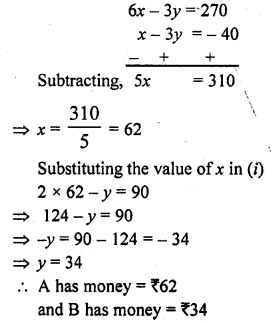Question 6 : ABCD is a cyclic quadrilateral such that ∠A = (4y + 20)°, ∠B = (3y – 5)°, ∠C = (+4x)° and ∠D = (7x + 5)°. Find the four angles. [NCERT]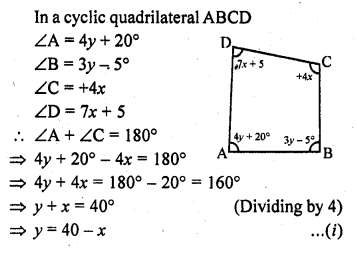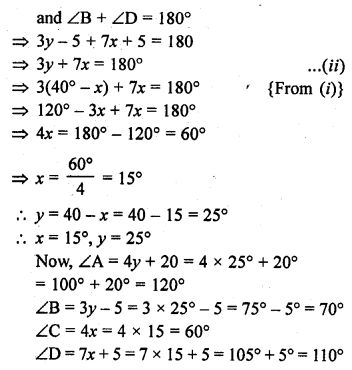Question 7 : 2 men and 7 boys can do a piece of work in 4 days. The same work is done in 3 days by 4 men and 4 boys. How long would it take one man and one boy to do it ?

Answer 7 : Let one man can do a work is = x days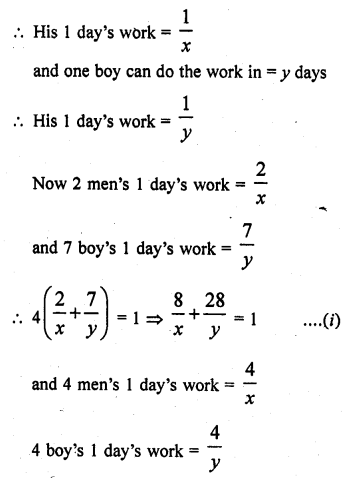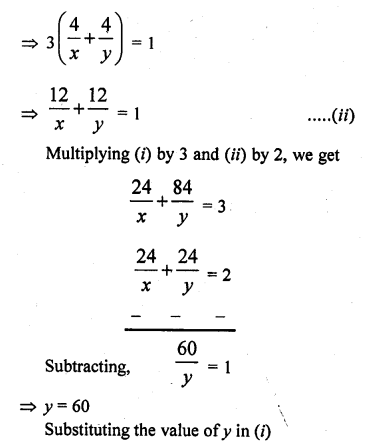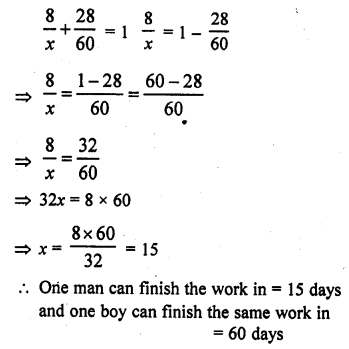Question 8 : In a ∆ABC, ∠A = x°, ∠B = (3x – 2)°, ∠C = y°. Also ∠C – ∠B = 9°. Find the three angles.

We know that sum of three angles of a triangle = 180°
∠A + ∠B + ∠C = 180°
=> x° + (3x – 2)° + y° = 180°
=> x + 3x – 2 + y = 180
=> 4x + y = 180 + 2 = 182 …(i)
∠C – ∠B = 9
y – (3x – 2) = 9
=> y – 3x + 2 = 9
=> y – 3x = 9 – 2
=> -3x + y = 7 ….(ii)
Subtracting (ii) from (i)
7x = 175 => x = 25
Substituting the value of x in (ii)
-3 x 25 + y = 7
=> -75 + y = 7
=> y = 7 + 75 = 82
∠A = x° = 25°
∠B = 3x – 2 = 3 x 25 – 2 = 73°
∠C = 7 = 82°

Question 9 : In a cyclic quadrilateral ABCD, ∠A = (2x + 4)°, ∠B = (y + 3)°, ∠C = (2y + 10)°, ∠D = (4x – 5)°. Find the four angles.

and ∠A = (2x + 4)°, ∠B = (y + 3)°, ∠C = (2y + 10)°, ∠D = (4x – 5)°
The sum of opposite angles = 180°
∠A + ∠C=180° and ∠B + ∠D = 180°
=> 2x + 4 + 2y + 10 = 180°
=> 2x + 2y + 14 = 180°
=> 2x + 2y = 180 – 14 = 166
=> x + y = 83 ….(i)
(Dividing by 2)
and y + 3 + 4x – 5 = 180°
=> 4x + 7 – 2 = 180°
4x + 7 = 180°+ 2= 182° ….(ii)
Subtracting (i) from (ii)
3x = 99
=> x = 33
Substituting the value of x in (i)
33 + y = 83
=> y = 83 – 33 = 50
∠A = 2x + 4 = 2 x 33 + 4 = 66 + 4 = 70°
∠B = y + 3 = 50 + 3 = 53°
∠C = 2y + 10 = 2 x 50 + 10 = 100 + 10 = 110°
∠D = 4x – 5 = 4 x 33 – 5 = 132 – 5 = 127°

Question 10 : Yash scored 40 marks in a test, getting 3 marks for each right answer and losing 1 mark for each wrong answer. Had 4 marks been awarded for each correct answer and 2 marks been deducted for each incorrect answer, then Yash would have scored 50 marks. How many questions were there in the test ?

Let number of right answers questions = x
and number of wrong answers questions = y
Now according to the given conditions,
3x – y = 40 ….(i)
and 4x – 2y = 50
=> 2x – y = 25 ….(ii)
(Dividing by 2)
Subtracting (ii) from (i), x = 15
Substituting the value of x in (i)
3 x 15 – y = 40
=> 45 – y = 40
=> y = 45 – 40 = 5
x = 15 and y = 5
Total number of questions = 15 + 5 = 20

Todays Deals### RD Chapter 3- Pair of Linear Equations in Two Variables Ex-3.11 Contributorskrishan

Name:
Email:

# Latest News# 9000 interview questions in different categories